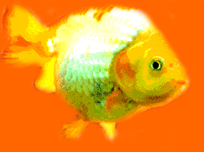//Author: Sophia Han

//Date: 05/12/02

//Description:  A virtual fish that swims around by default, eats when the switch is on, and dies when overfed

//draws the fish given the (x,y) coordinates of the center, and 0 or 1 to specify which direction to face

void fish(int x, int y, int dir){

canvas(0);

fill(1);

circle(x, y, 2);

if (dir == 0){

line((x-3), (y-1), (x-3), (y+1));

line((x-4), (y-2), (x-4), (y+2));

point((x-5), (y-2));

point((x-5), (y+2));

stroke(0);

point((x+1), (y-1));

stroke(1);

}

else{

line((x+3), (y-1), (x+3), (y+1));

line((x+4), (y-2), (x+4), (y+2));

point((x+5), (y-2));

point((x+5), (y+2));

stroke(0);

point((x-1), (y-1));

stroke(1);

}

}

//draws a fish facing upwards given the (x,y) coordinates of the center

void fishUp(int x, int y){

canvas(0);

fill(1);

stroke(1);

circle(x, y, 2);

line((x-1), (y+3), (x+1), (y+3));

line((x-2), (y+4), (x+2), (y+4));

point((x-2), (y+5));

point((x+2), (y+5));

stroke(0);

point((x+1), (y-1));

}

//moves the fish to the top of the display, moves the mouth to simulate eating, and returns the fish to

//its original position

void eat(int y){

int moveUp = y;

int startPos = y;

int mouth;

while(moveUp > 2){

moveUp = moveUp - 1;

fishUp(5, (moveUp));

pause(30);

}

for (mouth = 0; mouth < 4; mouth++){

fishUp(5, 2);

stroke(0);

line(4, 0, 4, 1);

pause(50);

stroke(1);

}

while(moveUp < startPos){

moveUp = moveUp + 1;

fish(4, moveUp, 1);

pause(30);

}

}

//shows the fish belly up, floating on the top of the display

void die()

{

while(true){

fish(3, 2, 1);

point(2, 1);

pause(50);

fish(2, 2, 1);

point(1, 1);

pause(50);

}

}

//checks to see if the switch is turned on (fish food touching the bowl), and reponds accordingly

int cycle(int x, int y, int dir, int feed){

if (feed == 1){

fish(x, y, dir);

pause(30);

return 0;

}

else{

:0 = 1;

pause(100);

:1 = 1;

pause(100);

:0 = 0;

:2 = 1;

pause(100);

:1 = 0;

:2 = 0;

eat(y);

return 1;

}

}

int feed = @2;

int death = 0;

while(death < 3){

death = 0;

feed = @2;

death = death + cycle(4, 5, 1, feed);

feed = @2;

death = death + cycle(4, 6, 1, feed);

feed = @2;

death = death + cycle(4, 7, 1, feed);

feed = @2;

death = death + cycle(4, 8, 1, feed);

feed = @2;

death = death + cycle(5, 8, 0, feed);

feed = @2;

death = death + cycle(5, 9, 0, feed);

feed = @2;

death = death + cycle(6, 10, 0, feed);

feed = @2;

death = death + cycle(7, 11, 0, feed);

feed = @2;

death = death + cycle(4, 9, 1, feed);

feed = @2;

death = death + cycle(4, 8, 1, feed);

feed = @2;

death = death + cycle(5, 7, 0, feed);

feed = @2;

death = death + cycle(5, 6, 0, feed);

feed = @2;

death = death + cycle(5, 5, 0, feed);

feed = @2;

death = death + cycle(4, 5, 1, feed);

feed = @2;

death = death + cycle(3, 4, 1, feed);

}

die();

MAS.110 Spring 2002